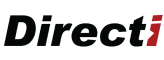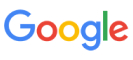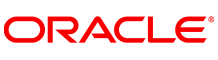New update is available. Click here to update.
Topics

# Jumping Numbers

Moderate0/80
Average time to solve is 15m+5 more companies

## Problem statement

You are given a positive integer N, your task is to find all the Jumping Numbers smaller than or equal to N.

A number is defined as a Jumping Number if all adjacent digits in it have an absolute difference of 1.

Example :
``````2, 23, and 4343456 are Jumping numbers but 296 and 89498 are not.
``````
Note:
``````The difference between ‘9’ and ‘0’ is not considered as 1. All single-digit numbers are considered as Jumping Numbers.
``````
Detailed explanation ( Input/output format, Notes, Images )
Constraints:
``````1 <= T <= 100
1 <= N <= 10^8

Time Limit: 1 sec
``````
Sample Input 1:
``````1
20
``````
Sample Output 1:
``````0 1 2 3 4 5 6 7 8 9 10 12
``````
Explanation For Sample Input 1:
``````These are all the jumping numbers from 0 to 12 as all the single-digit numbers are jumping numbers and out of the two-digit numbers only 10 and 12 are the jumping numbers less than 20 as the absolute difference in the adjacent digits of 10 and 12 is 1.
``````
Sample Input 2:
``````2
1
30
``````
Sample Output 2:
``````0 1
0 1 2 3 4 5 6 7 8 9 10 12 21 23
``````Console